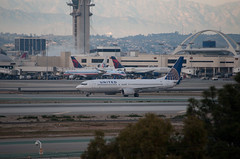## Mini Case: Will Leasing Fly at Continental?CFM 3 Ch 21 Minicase Will Leasing Fly at Continental? in \$28 only

1. Calculate the net advantage to leasing, using the expected residual value and assuming Continental can use all the tax benefits of ownership with a tax rate of 40% and straight line depreciation to the expected residual value. Assume that Continental issues 80% secured debt and 20% unsecured debt to finance a purchase.

a) Calculate rt–the project cost of capital.

b) Calculate the expected lease residual value per aircraft.

c) Calculate the quarterly CFAT per aircraft under the leasing option.

• Hint: It should be the same each quarter hroughout the term of the lease.
• The lease payment is tax deductible.
• Under the leasing option Continental forgoes the depreciation tax deduction.

d) Calculate the NAL.

• Assume quarterly compounding to match the lease payments.
• Continental’s required return on the asset—r, is given.
• Assume no incremental difference in operating expenses between the purchasing and leasing options.
• Assume that the lessor claims the ITC.

2.  Calculate the net advantage to leasing, assuming Continental cannot use any of the tax benefits of ownership and the residual value is (i) the expected residual value, (ii) \$50 million, and (iii) \$10 million

1. The amount of money borrowed or invested is called the maturity value.
True
False

2. When solving a simple interest problem, the rate should be written as a decimal number.
True
False

3. The interest paid on a \$10,000.00 loan for 2 years at 13.5% interest is \$1,250.00.
True
False

4. The formula to find the rate is interest divided by (principal times time).
True
False

5. 8% for 45 days is equal to 0.01.
True
False